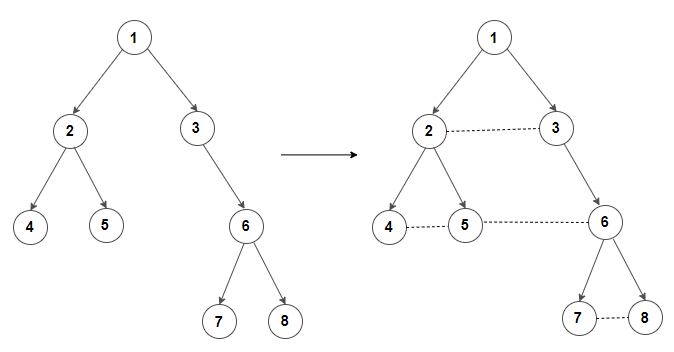# How To Link nodes present in each level of a binary tree in the form of a linked list?

Given a binary tree, write an efficient algorithm to link nodes at the same level in the form of a linked list like structure.

For example, convert binary tree to the left to binary tree to rightWe can solve this problem in linear time by using Hashing. The idea is to traverse the tree in preorder fashion and store nodes present at each level in a map from left to right. Then after every node is proccessed, we iterate through the map and for each level, we set next node for every node present in it.
Here’s a C++ program that demonstrates it:

Output:

1 -> null
2 -> 3 -> null
4 -> 5 -> 6 -> null
7 -> 8 -> null

The time complexity of above solution is O(n) and auxiliary space used by the program is O(n) for the unordered map.

### How can we solve this using constant space?

The idea is to traverse the tree in preorder fashion and ensure that all nodes at the current level is linked before all nodes at the next level i.e. next pointer of the parent nodes is set before its children.
Then we update the next pointer of parent’s left child to parent’s right child. If right child doesn’t exist, we link parent’s left child to first node in the next level. Similarly, we update the next pointer of parent’s right child to first node in the next level. If we follow this recursively for left and right subtrees, we will end up having connected nodes at each level.

Output:

1 -> null
2 -> 3 -> null
4 -> 5 -> 6 -> null
7 -> 8 -> null

The time complexity of above solution is O(n2) and takes implicit space for the call stack. We can easily convert above program to non-recursive one which takes O(1) space. The iterative version can be seen here.